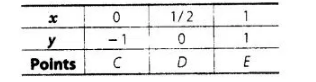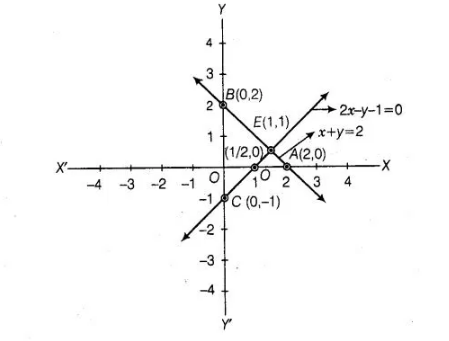# Draw the graph of the pair of equations 2x + y= 4

Question:

Draw the graph of the pair of equations 2x + y= 4 and 2x – y = 4. Write the vertices of the triangle formed by these lines and the y-axis, find the area of

this triangle? ’

Solution:

The given pair of llnear equations Table for line $2 x+y=4$,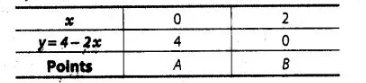and table for line $2 x-y=4$,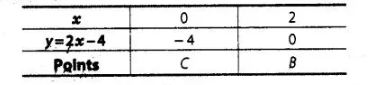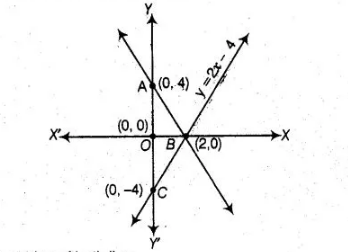Graphical representation of both lines.

Here, both lines and $Y$-axis form a $\triangle A B C$.

Hence, the vertices of a $\triangle A B C$ are $A(0,4) B(2,0)$ and $C(0,-4)$.

$\therefore \quad$ Required area of $\triangle A B C=2 \times$ Area of $\triangle A O B$

$=2 \times \frac{1}{2} \times 4 \times 2=8$ sq units

Hence, the required area of the triangle is 8 sq units.

If $x=0$, then $y=-1$; if $x=\frac{1}{2}$, then $y=0$ and if $x=1$, then $y=1$